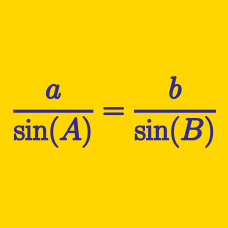Geometry

# Sine Rule - Ambiguous Case

How many distinct triangles are there such that $a=6, c=15, \angle A=30^\circ?$

Note: $$a, b$$ and $$c$$ are the lengths of the sides opposite the vertices $$A,$$ $$B$$ and $$C,$$ respectively.

How many distinct triangles are there such that $a=20, c=16, \angle A=30^\circ?$

Note: $$a, b$$ and $$c$$ are the lengths of the sides opposite the vertices $$A,$$ $$B$$ and $$C,$$ respectively.

How many distinct triangles are there such that $\angle A=30^\circ, a=9, b=9\sqrt{3} ?$

Note: $$a, b$$ and $$c$$ are the lengths of the sides opposite the vertices $$A,$$ $$B$$ and $$C,$$ respectively.

In triangle $$ABC$$, $$a=13\sqrt{3}$$, $$b=13$$, $$\angle B=30^{\circ}$$, and $$\angle C$$ is acute. What is the value of $$\angle C$$ (in degrees)?

Details and assumptions

$$a$$, $$b$$ and $$c$$ are the lengths of the sides opposite to the vertices $$A$$, $$B$$ and $$C$$, respectively. An acute angle is an angle strictly less than $$90^\circ$$.

How many distinct triangles are there such that $a=35, b=56, \angle A=30^\circ?$

Note: $$a, b$$ and $$c$$ are the lengths of the sides opposite the vertices $$A,$$ $$B$$ and $$C,$$ respectively.

×

Problem Loading...

Note Loading...

Set Loading...